# Ratio + circle - math problems

#### Number of problems found: 33

• Circumference - a simple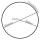What is the ratio of the circumference of any circle and its diameter? Write the result as a real number rounded to 2 decimal places.
• CirclesThe areas of the two circles are in the ratio 2:20. The larger circle has a diameter 20. Calculate the radius of the smaller circle.
• Ratio of squaresA circle is given in which a square is inscribed. The smaller square is inscribed in a circular arc formed by the side of the square and the arc of the circle. What is the ratio of the areas of the large and small squares?
• Rectangle - desc circle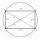Length of the sides of the rectangle are at a ratio 1: 3 . Radius of the circle circumscribed to rectangle is 10 cm. Calculate the rectangle's perimeter.
• Ratio of sidesCalculate the area of a circle with the same circumference as the circumference of the rectangle inscribed with a circle with a radius of r 9 cm so that its sides are in ratio 2 to 7.
• Three shapes1/5 of a circle is shaded. The ratio of area if square to the sum of area of rectangle and that of the circle is 1:2. 60% of the square is shaded and 1/3 of the rectangle is shaded. What is the ratio of the area of circle to that of the rectangle?
• Triangle in circle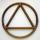Vertices of the triangle ABC lies on a circle with radius 3 so that it is divided into three parts in the ratio 4:4:4. Calculate the circumference of the triangle ABC.
• Ratio of volumesIf the heights of two cylindrical drums are in the ratio 7:8 and their base radii are in the ratio 4:3. What is the ratio of their volumes?
• Arc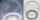The length of the circle is 41 amd arc length of the circle 9. What is the magnitude of the angle of this arc?
• Circumferential angleVertices of the triangle ΔABC lies on circle and divided it into arcs in the ratio 2:2:9. Determine the size of the angles of the triangle ΔABC.
• ArcCircle arc corresponding to angle is 32° is 28 dm long. What is the length of the entire circle?
• Arc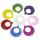What area of a circle occupied the flowers planted in the arc of a circle with radius 4 m with central angle 45°?
• Circle sectionEquilateral triangle with side 33 is inscribed circle section whose center is in one of the vertices of the triangle and the arc touches the opposite side. Calculate: a) the length of the arc b) the ratio betewwn the circumference to the circle sector and
• Inscribed triangle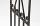To a circle is inscribed triangle so that the it's vertexes divide circle into 3 arcs. The length of the arcs are in the ratio 2:3:7. Determine the interior angles of a triangle.
• Sphere cutsAt what distance from the center intersects sphere with radius R = 56 plane, if the cut area and area of the main sphere circle is in ratio 1/2.
• InfinityIn a square with side 19 is inscribed circle, the circle is inscribed next square, again circle, and so on to infinity. Calculate the sum of the area of all these squares.
• Velocity ratio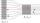Determine the ratio at which the fluid velocity in different parts of the pipeline (one part has a diameter of 5 cm and the other has a diameter of 3 cm), when you know that at every point of the liquid is the product of the area of tube [S] and the fluid
• Sphere in coneA sphere is inscribed in the cone (the intersection of their boundaries consists of a circle and one point). The ratio of the surface of the ball and the contents of the base is 4: 3. A plane passing through the axis of a cone cuts the cone in an isoscele
• Axial sectionAxial section of the cylinder has a diagonal 40 cm. The size of the shell and the base surface are in the ratio 3:2. Calculate the volume and surface area of this cylinder.
• Cylinder surface, volumeThe area of the cylinder surface and the cylinder jacket are in the ratio 3: 5. The height of the cylinder is 5 cm shorter than the radius of the base. Calculate surface area and volume of the cylinder.

Do you have an interesting mathematical word problem that you can't solve it? Submit a math problem, and we can try to solve it.

We will send a solution to your e-mail address. Solved examples are also published here. Please enter the e-mail correctly and check whether you don't have a full mailbox.

Please do not submit problems from current active competitions such as Mathematical Olympiad, correspondence seminars etc...

Check out our ratio calculator. Ratio - math word problems. Circle Problems.